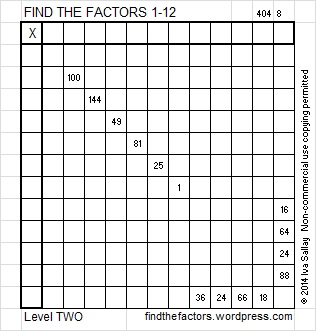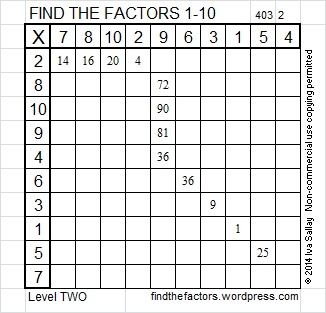# 46 and South East Arrow Puzzle

46 is a composite number. 46 = 1 x 46 or 2 x 23. Factors of 46: 1, 2, 23, 46. Prime factorization: 46 = 2 x 23.46 is never a clue in the FIND THE FACTORS puzzles.

Wikipedia has a chart of 116 different directional arrow symbols. Each one of them has a name. Today’s puzzle represents the South East Arrow.To solve the puzzle write the numbers 1 -12 in the top row and again in the first column so that the numbers you write and the clues in the puzzle work together to make a multiplication table.

This week’s puzzles are available in an excel file here. If you have a spreadsheet program on your computer, you can access it. If you enable editing in excel, you can type your answers directly onto the puzzle, and you can also easily print the puzzles.

Here are the factors of last week’s hook-shaped level 2 puzzle: